# RD Sharma Class 9 Solutions Chapter 1 Number Systems Ex 1.1

## RD Sharma Class 9 Solutions Chapter 1 Number Systems Ex 1.1

These Solutions are part of RD Sharma Class 9 Solutions. Here we have given RD Sharma Class 9 Solutions Chapter 1 Number Systems Ex 1.1

Question 1.
Is zero a rational number? Can you write it P in the form $$\frac { p }{ q }$$ , where p and q are integers and q ≠ 0? [NCERT]
Solution:
Yes, zero is a rational number e.g.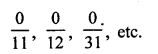Question 2.
Find five rational numbers between 1 and 2. [NCERT]
Solution:
We know that one rational number between two numbers a and b = $$\frac { a+b }{ 2 }$$
Therefore one rational number between 1 and 2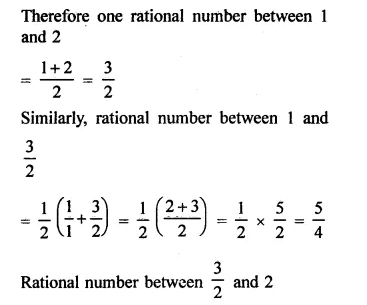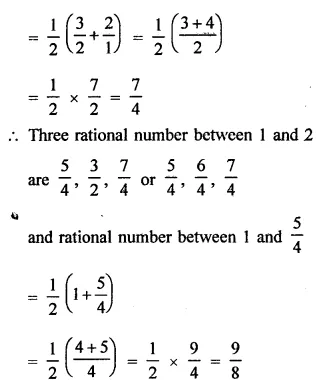Question 3.
Find six rational numbers between 3 and 4. [NCERT]
Solution:
One rational number between 3 and 4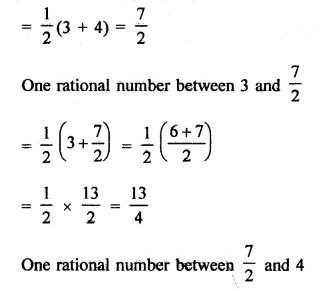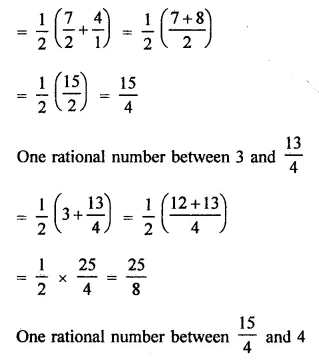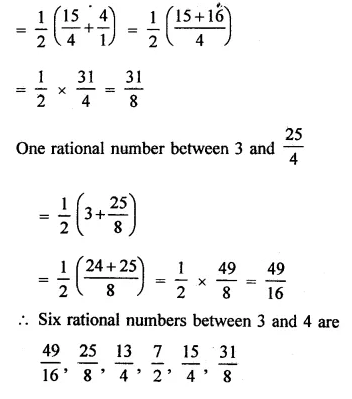Question 4.
Find five rational numbers between $$\frac { 3 }{ 5 }$$ and $$\frac { 4 }{ 5 }$$
Solution: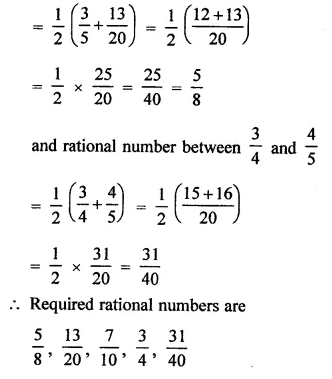Question 5.
Are the following statements true or false?
(i) Every whole number is a natural number. [NCERT]
(ii) Every integer is a rational number.
(iii) Every rational number is an integer.
(iv) Every natural number is a whole number,
(v) Every integer is a whole number.
(vi) Every rational number is a whole number.
Solution:
(i) False, as 0 is not a natural number.
(ii) True.
(iii) False, as $$\frac { 1 }{ 2 }$$, $$\frac { 1 }{ 3 }$$ etc. are not integers.
(iv) True.
(v) False, ∵ negative natural numbers are not whole numbers.
(vi) False, ∵ proper fraction are not whole numbers

Hope given RD Sharma Class 9 Solutions Chapter 1 Number Systems Ex 1.1 are helpful to complete your math homework.

If you have any doubts, please comment below. Learn Insta try to provide online math tutoring for you.